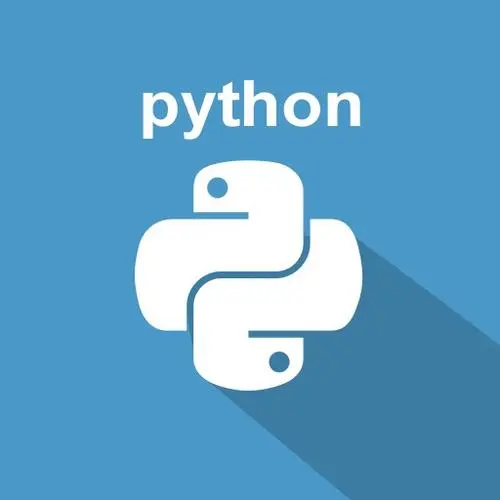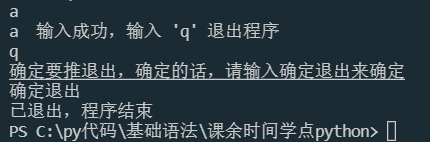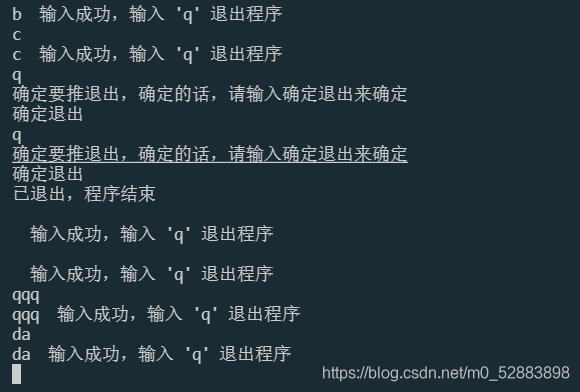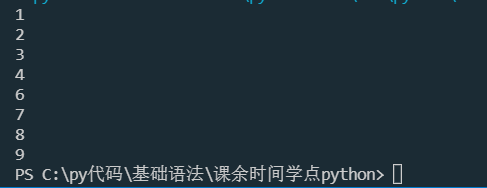# 趁着课余时间学点 Python（六）终止循环，阻断循环ベ布小禅@[TOC]

# 条件控制语句的补充

## break

break 的作用是打破结构体，使程序退出当前代码块

while True:    a = input()    if a == "q":        break    else:        print(a + "  输入成功，输入 'q' 退出程序")while True:    a = input()    if a == "q":        print("确定要推退出，确定的话，请输入确定退出来确定")        if input() == "确定退出":            print("已退出，程序结束")        break    else:        print(a + "  输入成功，输入 'q' 退出程序")## continue

continue 是跳出当前循环，执行下一循环，也就是说，他并没有令循环终止的能力，他只能令循环少循环一些次数

while True:    a = input()    if a == "q":        print("确定要推退出，确定的话，请输入确定退出来确定")        if input() == "确定退出":            print("已退出，程序结束")            else:        print(a + "  输入成功，输入 'q' 退出程序")for i in range(10):    if i % 5 == 0:        continue    else:        print(i)## return

return 意为返回，是用在函数中的返回值的，至于函数是什么，我们会在本专栏中陆续讲述，你先看效果就可以了

def sum(a, b):    for i in range(10):        if i<a:            pass        else:            a+=b            return aprint(sum(5, 2))

pass 的意思就相当于什么都不做

def sum(a, b):    for i in range(10):        if i<a:            pass        else:            a+=b        return aprint(sum(5, 2))

def sum(a, b):    for i in range(10):        if i<a:            pass        else:            a+=b    return aprint(sum(5, 2))

# 结语## 评论